# idFeedforwardNetwork

Multilayer feedforward neural network mapping function for nonlinear ARX models (requires Deep Learning Toolbox)

## Description

An `idFeedforwardNetwork` object implements a neural network function, and is a nonlinear mapping object for estimating nonlinear ARX models. This mapping object lets you use `network` (Deep Learning Toolbox) objects that are created using Deep Learning Toolbox™ in nonlinear ARX models.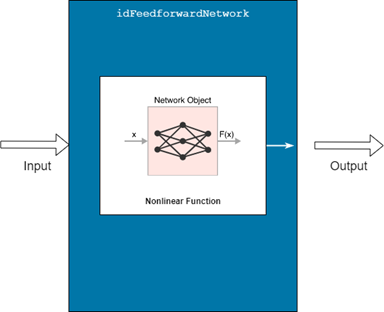Mathematically, `idFeedforwardNetwork` is a function that maps m inputs X(t) = [x(t1),x2(t),…,xm(t)]T to a scalar output y(t), using a multilayer feedforward (static) neural network, as defined in Deep Learning Toolbox.

You create multi-layer feedforward neural networks by using commands such as `feedforwardnet` (Deep Learning Toolbox), `cascadeforwardnet` (Deep Learning Toolbox) and `linearlayer` (Deep Learning Toolbox). When you create the network,

• Designate the input and output sizes to be unknown by leaving them at the default value of zero (recommended method). When estimating a nonlinear ARX model using the `nlarx` command, the software automatically determines the input and output sizes of the network.

• Initialize the sizes manually by setting input and output ranges to m-by-2 and 1-by-2 matrices, respectively, where m is the number of nonlinear ARX model regressors and the range values are minimum and maximum values of regressors and output data, respectively.

Use `evaluate(net_estimator,x)` to compute the value of the function defined by the `idFeedforwardNetwork` object `net_estimator` at input value x. When used for nonlinear ARX model estimation, x represents the model regressors for the output for which the `idFeedforwardNetwork` object is assigned as the nonlinearity estimator.

You cannot use `idFeedforwardNetwork` when the `Focus` option in `nlarxOptions` is `'simulation'` because the underlying `network` object is considered to be nondifferentiable for estimation. Minimization of simulation error requires differentiable nonlinear functions.

Use `idFeedforwardNetwork` as the value of the `OutputFcn` property of an `idnlarx` model. For example, specify `idFeedforwardNetwork` when you estimate an `idnlarx` model with the following command.

`sys = nlarx(data,regressors,idFeedforwardNetwork)`
When `nlarx` estimates the model, it essentially estimates the parameters of the `idFeedforwardNetwork` function.

## Creation

### Syntax

``net_estimator = idFeedforwardNetwork(Network)``

### Description

example

````net_estimator = idFeedforwardNetwork(Network)` creates a feedforward neural network mapping object that is based on the feedforward (static) network object `Network` that has been created using one of the neural network commands `feedforwardnet`, `cascadeforwardnet`, or `linearlayer`. `Network` must represent a static mapping between the inputs and output without I/O delays or feedback. The number of outputs of the network, if assigned, must be set to one. For a multiple-output nonlinear ARX models, create a separate `idFeedforwardNetwork` object for each output—that is, each element of the output function must represent a single-output network object.```

## Properties

expand all

Feedforward neural network object, typically created using `feedforwardnet` (Deep Learning Toolbox), `cascadeforwardnet` (Deep Learning Toolbox) or `linearlayer` (Deep Learning Toolbox).

Input signal names for the inputs to the mapping object, specified as a 1-by-m cell array, where m is the number of input signals. This property is determined during estimation.

Output signal name for the output of the mapping object, specified as a 1-by-1 cell array. This property is determined during estimation.

Option to train the neural network, specified as `true` or `false`. Set `Free` to false when the neural network you are using has already been trained and is known to provide good fit results. The `Free` property is especially useful when your idnlarx model has multiple outputs that each use a neural network. Setting `Free` to `false` for well trained networks allows processing time to be focused on the networks that do need training.

## Examples

collapse all

Create a neural network mapping object that uses a feedforward neural network with three hidden layers, transfer functions of types `logsig`, `radbas`,and `purelin`, and unknown input and output sizes.

Create a neural network.

```net = feedforwardnet([4 6 1]); net.layers{1}.transferFcn = 'logsig'; net.layers{2}.transferFcn = 'radbas'; net.layers{3}.transferFcn = 'purelin'; ```

View the network diagram.

```view(net) ```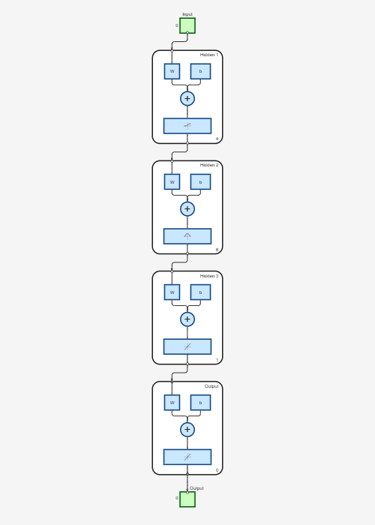Create a neural network mapping object.

```net_estimator = idFeedforwardNetwork(net); ```

Create a single-layer, cascade-forward network with unknown input and output sizes and use this network for nonlinear ARX model estimation.

Create a cascade-forward neural network with 20 neurons and unknown input/output sizes.

`net = cascadeforwardnet(20);`

Create a feedforward neural network mapping object.

`net_estimator = idFeedforwardNetwork(net);`

Load the estimation data.

```load twotankdata data = iddata(y,u,0.2);```

Estimate a nonlinear ARX model `sys`.

`sys = nlarx(data,[2 2 1],net_estimator);`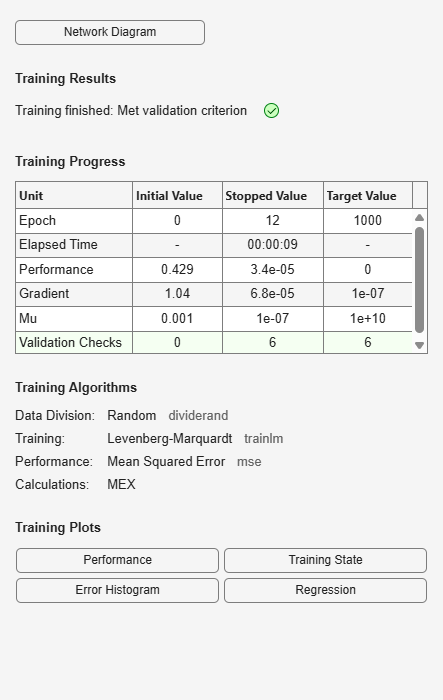Compare the model response to the measured output signal.

`compare(data,sys)`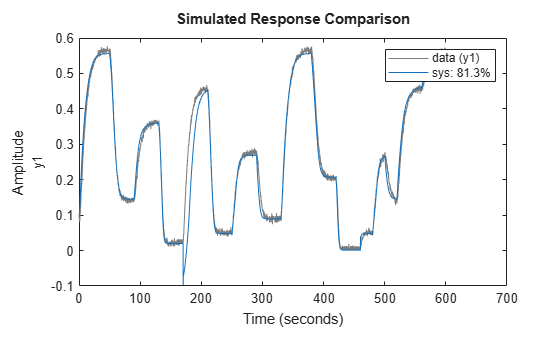The plot shows good agreement between the measured signal and the simulated model output signal.

Initialize the input-output sizes of a two-layer feed-forward neural network based on estimation data, and use this network for nonlinear ARX estimation.

```load iddata7 z7 z7 = z7(1:200);```

Create a template nonlinear ARX model with no nonlinearity.

`model = idnlarx([4 4 4 1 1],[]);`

This model has six regressors and is used to define the regressors. The range of regressor values for input-output data in `z7` is then used to set the input ranges in the neural network object, as shown in the next steps.

Obtain the model regressor values.

```R = getreg(model,'all',z7); R = R.Variables;```

Create a two-layer, feed-forward neural network and initialize the network input and output dimensions to 2 and 1, respectively. Use 5 neurons for first layer and 7 for second layer.

`net = feedforwardnet([5 7]);`

Determine input range.

`InputRange = [min(R);max(R)].';`

Initialize input dimensions of estimator.

`net.inputs{1}.range = InputRange;`

Determine output range.

`OutputRange = [min(z7.OutputData),max(z7.OutputData)];`

Initialize output dimensions of estimator and the choice of training function.

```net.outputs{net.outputConnect}.range = OutputRange; net.trainFcn = 'trainbfg';```

Create a neural network nonlinearity estimator.

`net_estimator = idFeedforwardNetwork(net);`

Specify the nonlinearity estimator in the model.

`model.Nonlinearity = net_estimator;`

Estimate the parameters of the network to minimize the prediction error between data and model. Estimate model.

`model = nlarx(z7,model);`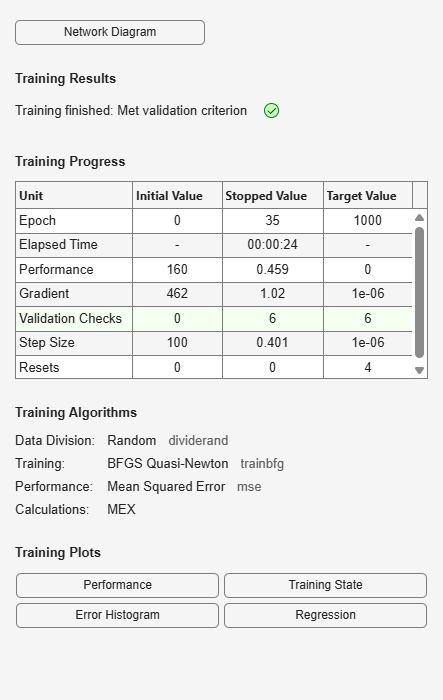Compare model's predicted response to measured output signal.

`compare(z7(1:100),model,1)`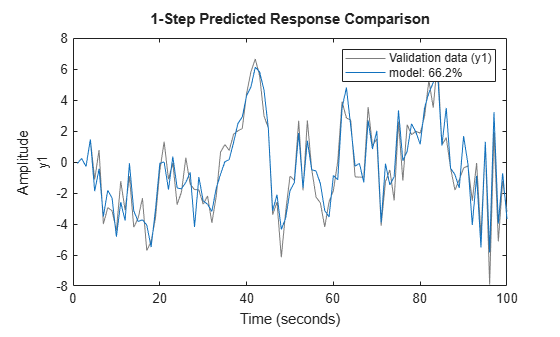## Algorithms

The `nlarx` command uses the `train` method of the `network` object, defined in the Deep Learning Toolbox software, to compute the network parameter values.

## Version History

Introduced in R2007a

expand all